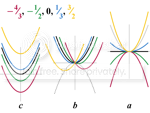0

## The Wolfram|Alpha Trick

Published on Thursday, July 07, 2011 in , , , , ,Ever do one of those tricks where you have someone pick a number, and have them do things like add 5, double it, subtract 4, divide by 2, subtract their original number, and you determine that the answer they got was 3?

As many people realize when you're doing such a trick, the whole process boils down to an algebraic formula that applies to any number. Today, we're going to turn that process on its head by figuring out a formula secretly chosen by your audience!

After being shown the following math trick by W. W. Sawyer, Martin Gardner wrote it up in his books, New Mathematical Diversions and The Colossal Book of Mathematics.

Here's the original write-up, in Martin Gardner's words:

Instead of asking someone to “think of a number” you ask him to “think of a formula.” To make the trick easy, it should be a quadratic formula (a formula containing no powers of x greater than x2). Suppose that he thinks of 5x2 + 3x - 7. While your back is turned so that you cannot see his calculations, ask him to subtitute 0, 1, and 2 for x, then tell you the three values that result for the entire expression. The values he gives you are -7, 1, 19. After a bit of scribbling (with practice you can do it in your head) you tell him the original formula!

The method is simple. Jot down in a row the value he gives to you. In a row beneath write the differences between adjacent pairs of numbers, always subtracting the number on the left from its neighbor on the right. In a third row put the difference between the numbers above it. The chart will look like this:The coefficient of x2, in the thought-of formula, is always half the bottom number of the chart. The coefficient of x is obtained by taking half the bottom number from the first number of the middle row. And the constant in the formula is simply the first number of the top row.

When this was originally published in the 1960s, I can't imagine this trick seeing much action outside of college math classes. You probably wouldn't expect that to change.

As so often happens, technology is at the point now where this trick is actually feasible for a much wider audience. If you have a pen or pencil, some paper, and a volunteer with a smartphone or tablet connected to the internet, you can do this trick just about anywhere, even for people who don't do math! The use of a mobile internet device means you don't have to turn your back on your audience at any time, since they can just hold the device facing themselves.

You could begin by talking about algebraic tricks like the one at the beginning of this post, and then explain that you're going to attempt to find a formula they create, instead.

Have them connect to www.wolframalpha.com (Wolfram|Alpha). If you're not already familiar with it, here's a video introduction to it by the inventor, Stephen Wolfram (Part 2 is here).

Start by asking your volunteer to choose a number from 1 to 20, and asking him to enter that number into the Wolfram|Alpha search box followed by xx. For example, if he chose 5, he would enter 5xx.

(Side note: Yes, Wolfram|Alpha will also take x^2 to mean the same thing, but entering xx only requires two characters to enter and doesn't slow down the presentation while they hunt for the ^ character.)

Next, ask them to enter a plus or minus symbol, followed by a different number of their choice from 1 to 20, followed by a single x this time. Finally, ask them to choose a plus or minus symbol again, ending with one more different number from 1 to 20.

Let's say they entered the equation from Martin Gardner's example above, as 5xx + 3x - 7. Tell them to click the = button on the Wolfram|Alpha search engine, where they should see something like this. Explain that, at this point, they should see graphs of the equation, along with things such as alternate forms of the equations, derivatives, and more!

Having them see all this information actually achieves two important things. First, it gives a visually dramatic idea of the complexity of the equation they've entered, making this feat seem much harder. Second, it also lets you know that they've entered the formula correctly, without you having to see it.

Wolfram|Alpha also makes the substitution part of the trick easy. Have them go back up to the search bar, making sure not to erase any part of the original equation they entered, and place the cursor after the equation. By having them add ,x=0 (note the comma) and clicking equals again, they'll see a much simpler page like this.

Have them give you the resulting answer, and then to do the same for x=1 and for x=2. Write them down, and figure out the original formula in the manner described above by Martin Gardner.

As you can see, the development of the internet, mobile wireless internet devices, and Wolfram|Alpha make a big difference in your potential audience for this math trick.

The internet even makes practicing this trick easy. You can use sites like random.org to generate random practice numbers from which to work out an equation, and use Wolfram|Alpha to see if you're right. You should stick to number sets where the leftmost and rightmost numbers are both odd or both even, so that you'll always be working with whole numbers.

If you're curious about the principle behind this routine, check out the Martin Gardner books I mentioned. Over in the Mental Gym, the same principle is used to determine a bill's serial number.